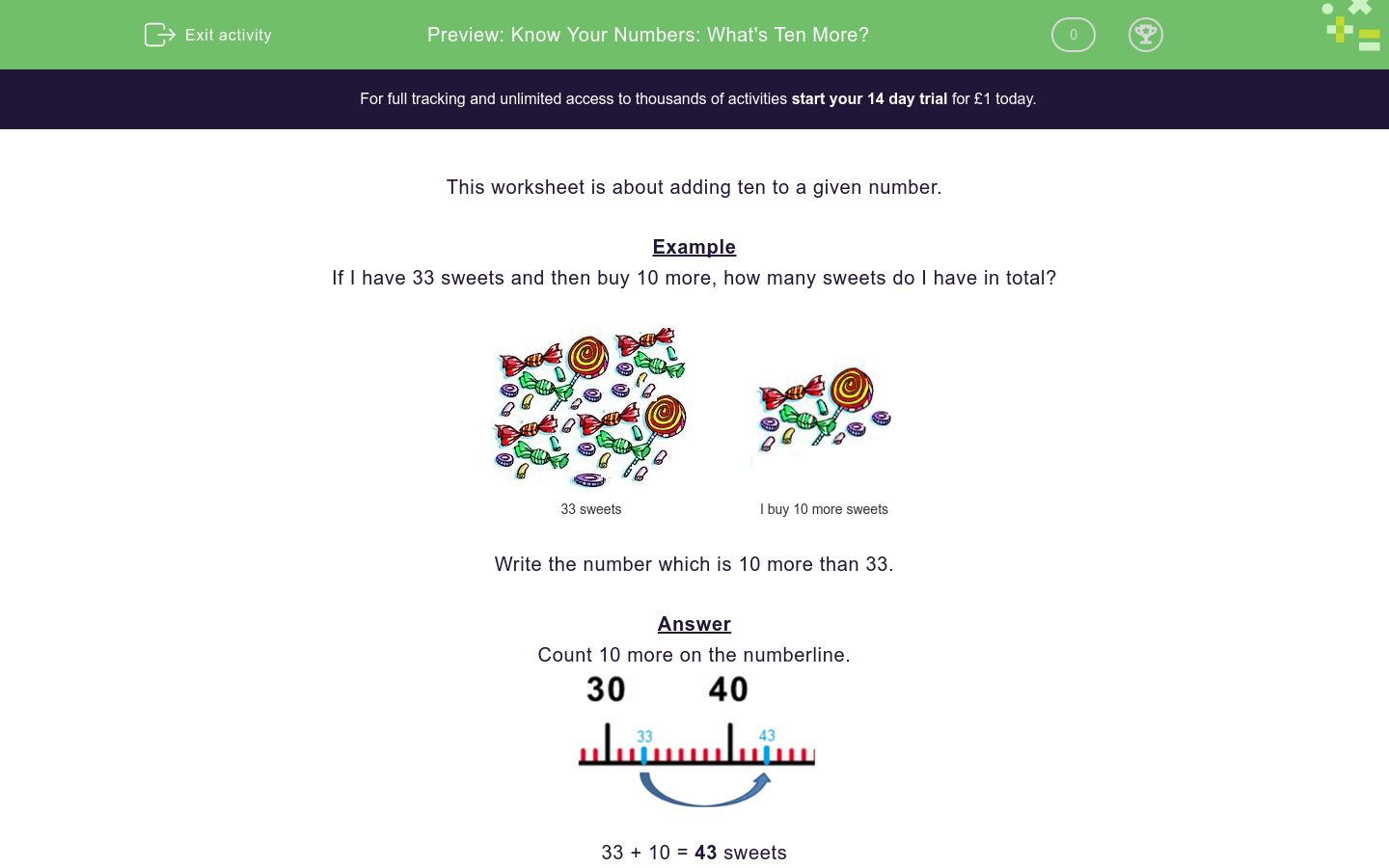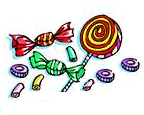# Know Your Numbers: What's Ten More?

In this worksheet, students add 10 to a given number (from a range between 0 and 100).Key stage:  KS 1

Curriculum topic:   Number: Number and Place Value

Curriculum subtopic:   Count in Steps (2, 3, 5 and 10)

Difficulty level:### QUESTION 1 of 10

Example

If I have 33 sweets and then buy 10 more, how many sweets do I have in total?33 sweets I buy 10 more sweets

Write the number which is 10 more than 33.

Count 10 more on the numberline.33 + 10 = 43 sweets

What is the number ten more than the given number?48 sweets I buy 10 more sweetsWrite the number which is 10 more than 48.

What is the number ten more than the given number?21 sweets I buy 10 more sweetsWrite the number which is 10 more than 21.

What is the number ten more than the given number?54 sweets I buy 10 more sweetsWrite the number which is 10 more than 54.

What is the number ten more than the given number?58 sweets I buy 10 more sweetsWrite the number which is 10 more than 58.

What is the number ten more than the given number?82 sweets I buy 10 more sweetsWrite the number which is 10 more than 82.

What is the number ten more than the given number?41 sweets I buy 10 more sweetsWrite the number which is 10 more than 41.

What is the number ten more than the given number?75 sweets I buy 10 more sweetsWrite the number which is 10 more than 75.

What is the number ten more than the given number?50 sweets I buy 10 more sweetsWrite the number which is 10 more than 50.

What is the number ten more than the given number?68 sweets I buy 10 more sweetsWrite the number which is 10 more than 68.

What is the number ten more than the given number?37 sweets I buy 10 more sweetsWrite the number which is 10 more than 37.

• Question 1

What is the number ten more than the given number?48 sweets I buy 10 more sweetsWrite the number which is 10 more than 48.

58
EDDIE SAYS

48+10=58

• Question 2

What is the number ten more than the given number?21 sweets I buy 10 more sweetsWrite the number which is 10 more than 21.

31
EDDIE SAYS

21+10=31

• Question 3

What is the number ten more than the given number?54 sweets I buy 10 more sweetsWrite the number which is 10 more than 54.

64
EDDIE SAYS

54+10=64

• Question 4

What is the number ten more than the given number?58 sweets I buy 10 more sweetsWrite the number which is 10 more than 58.

68
EDDIE SAYS

58+10=68

• Question 5

What is the number ten more than the given number?82 sweets I buy 10 more sweetsWrite the number which is 10 more than 82.

92
EDDIE SAYS

82+10=92

• Question 6

What is the number ten more than the given number?41 sweets I buy 10 more sweetsWrite the number which is 10 more than 41.

51
EDDIE SAYS

41+10=51

• Question 7

What is the number ten more than the given number?75 sweets I buy 10 more sweetsWrite the number which is 10 more than 75.

85
EDDIE SAYS

75+10=85

• Question 8

What is the number ten more than the given number?50 sweets I buy 10 more sweetsWrite the number which is 10 more than 50.

60
EDDIE SAYS

50+10=60

• Question 9

What is the number ten more than the given number?68 sweets I buy 10 more sweetsWrite the number which is 10 more than 68.

78
EDDIE SAYS

68+10=78

• Question 10

What is the number ten more than the given number?37 sweets I buy 10 more sweetsWrite the number which is 10 more than 37.

47
EDDIE SAYS

37+10=47

---- OR ----

Sign up for a £1 trial so you can track and measure your child's progress on this activity.

### What is EdPlace?

We're your National Curriculum aligned online education content provider helping each child succeed in English, maths and science from year 1 to GCSE. With an EdPlace account you’ll be able to track and measure progress, helping each child achieve their best. We build confidence and attainment by personalising each child’s learning at a level that suits them.

Get started